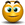# Using the regression equation

## Excel Help for Using The Regression Equation in Analyzing Corporate Financial DataThe view plot the predicted log(t) and p, simply use the calculated regression equation with the original input values. Excel Business Forums Administrator Posted by Excel Helper on 27 Sep 2010